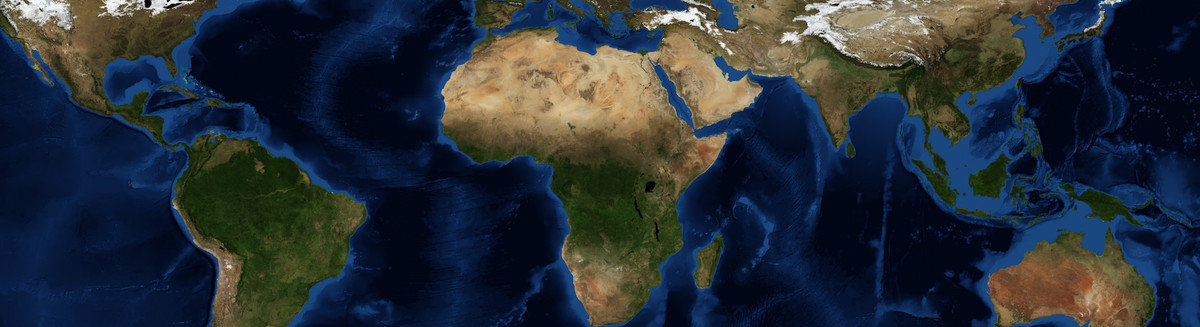This is a Preprint and has not been peer reviewed. The published version of this Preprint is available: https://doi.org/10.1007/s00477-018-1573-6. This is version 13 of this Preprint.

##### Abstract

The goal of quantile regression is to estimate conditional quantiles for specified values of quantile probability using linear or nonlinear regression equations. These estimates are prone to "quantile crossing", where regression predictions for different quantile probabilities do not increase as probability increases. In the context of the environmental sciences, this could, for example, lead to estimates of the magnitude of a 10-yr return period rainstorm that exceed the 20-yr storm, or similar nonphysical results. This problem, as well as the potential for overfitting, is exacerbated for small to moderate sample sizes and for nonlinear quantile regression models. As a remedy, this study introduces a novel nonlinear quantile regression model, the monotone composite quantile regression neural network (MCQRNN), that (1) simultaneously estimates multiple non-crossing, nonlinear conditional quantile functions; (2) allows for optional monotonicity, positivity/non-negativity, and generalized additive model constraints; and (3) can be adapted to estimate standard least-squares regression and non-crossing expectile regression functions. First, the MCQRNN model is evaluated on synthetic data from multiple functions and error distributions using Monte Carlo simulations. MCQRNN outperforms the benchmark models, especially for non-normal error distributions. Next, the MCQRNN model is applied to real-world climate data by estimating rainfall Intensity-Duration-Frequency (IDF) curves at locations in Canada. IDF curves summarize the relationship between the intensity and occurrence frequency of extreme rainfall over storm durations ranging from minutes to a day. Because annual maximum rainfall intensity is a non-negative quantity that should increase monotonically as the occurrence frequency and storm duration decrease, monotonicity and non-negativity constraints are key constraints in IDF curve estimation. In comparison to standard QRNN models, the ability of the MCQRNN model to incorporate these constraints, in addition to non-crossing, leads to more robust and realistic estimates of extreme rainfall.

##### DOI

https://doi.org/10.31223/osf.io/wg7sn

##### Subjects

Artificial Intelligence and Robotics, Climate, Computer Sciences, Earth Sciences, Hydrology, Oceanography and Atmospheric Sciences and Meteorology, Physical Sciences and Mathematics, Statistical Models, Statistics and Probability

##### Keywords

Precipitation, Quantile regression, R package, Neural Network, Climate Extremes, rainfall, Intensity-Duration-Frequency (IDF) curves, non-crossing quantile curves, predictive uncertainty, statistical model

##### Dates

Published: 2017-12-05 00:14

Last Updated: 2018-04-17 18:29